GFG App
Open AppBrowser
Continue

# Class 10 NCERT Solutions- Chapter 9 Some Application of Trigonometry – Exercise 9.1 | Set 1

### Question 1. A circus artist is climbing a 20 m long rope, which is tightly stretched and tied from the top of a vertical pole to the ground. Find the height of the pole, if the angle made by the rope with the ground level is 30° (see Fig.).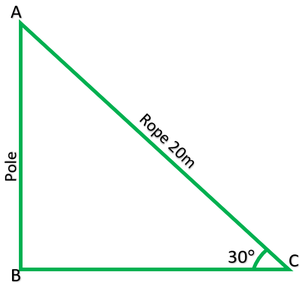Solution:

In rt ∆ABC,

AB = pole = ?

AC = rope = 20m

sinθ =sin30° =AB = 1/2 * 20

AB = 10m

Height of pole = 10m

### Question 2. A tree breaks due to storm and the broken part bends so that the top of the tree touches the ground making an angle 30° with it. The distance between the foot of the tree to the point where the top touches the ground is 8 m. Find the height of the tree.

Solution:

In rt ∆ABC,

BC = 8m= tan30°= 1/√3

AB = 8/√3          -(1)

Now,= cos30°

8/AC = √3/2

√3AC = 16

AC = 16/√3           -(2)

From (1) and (2)

Height of tree = AB + AC

= 8/√3 * 16√3

= 8√3 m

8 * 1.73 = 13.84m

The height of the tree is 13.84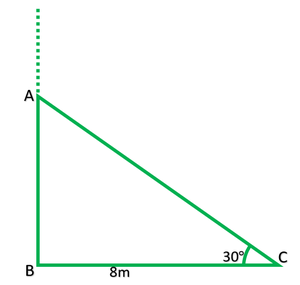### Question 3. A contractor plans to install two slides for the children to play in a park. For the children below the age of 5 years, she prefers to have a slide whose top is at a height of 1.5 m and is inclined at an angle of 30° to the ground, whereas for elder children, she wants to have a steep slide at a height of 3 m, and inclined at an angle of 60° to the ground. What should be the length of the slide in each case?

Solution:

In rt ∆ABC,

AB = 1.5m

AC = side = ?= sin30°

1.5/AC = 1/2

AC = 1/5 * 2

AC = 3m

In rt ∆PQR,

PQ = 3m

PR = side = ?= sin60°

3/PR = √3/2

√3 PR = 6

PR = 6/√3

6/√3 * √3/√3

= 2√3

= 2 * 1.73

= 3.46m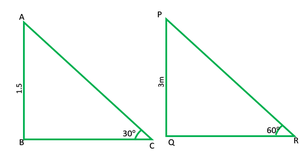### Question 4. The angle of elevation of the top of a tower from a point on the ground, which is 30 m away from the foot of the tower, is 30°. Find the height of the tower.

Solution:

In rt ∆ABC,

AB = tower = ?

BC = 30m= tan30°

AB/30 = 1/√3

AB = 30/√3

AB = 30/√3 * √3/√3

= (30√3)/3 = 10√3

= 10 * 1.73

= 17.3m

The height of tower 17.3m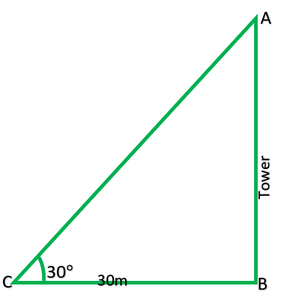### Question 5. A kite is flying at a height of 60 m above the ground. The string attached to the kite is temporarily tied to a point on the ground. The inclination of the string with the ground is 60°. Find the length of the string, assuming that there is no slack in the string.

Solution:

In rt ∆ABC,

AB = 6Om

AC = string = ?= sin60°

60/AC = √3/3

√3 AC = 60 * 2

AC = 120/120/(√3) * √3/√3

120/√3 * √3/√3

40 = √3

40 * 1.73 = 69.20m

Length of the string is 69.20m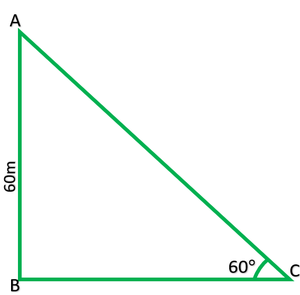### Question 6. A 1.5 m tall boy is standing at some distance from a 30 m tall building. The angle of elevation from his eyes to the top of the building increases from 30° to 60° as he walks towards the building. Find the distance he walked towards the building.

Solution:

In fig AB = AE – 1.5

= 30 – 1.5

= 28.5

In rt ∆ABD,= tan30°

= 28.5/BD = 1/√3

BD = 28.5√3           -(1)

In rt ∆ABC,= tan60°

28.5/BC*√3

√3 BC = 28.5

BC = 28.5/√3          -(2)

CD = BD − BC

= 28.5√3 – 28.5/√3

= 28.5(2/√3)

57/√3 * √3/√3 = (57√3)/3 = 19√3

19 * 1.73 = 32.87m

The boy walked 32.87m towards the building.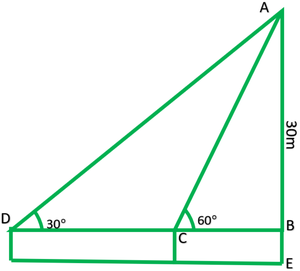### Question 7. From a point on the ground, the angles of elevation of the bottom and the top of a transmission tower fixed at the top of a 20 m high building are 45° and 60° respectively. Find the height of the tower.

Solution:

In fig:

AB = tower = ?

BC = building = 20m

In rt ∆BCD= tan45°

20/CD = 1/1

CD = 20

In rt. ∆ACD,= tan60°

AC/20 = √3/1

AC = 20√3           -(1)

AB = AC-BC

20√3 – 20

20(√3 – 1)

20(1.732 – 1)

20(0.732)

14.64m

The height of the tower is 14.6m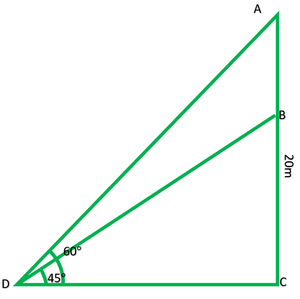### Question 8. A statue, 1.6 m tall, stands on the top of a pedestal. From a point on the ground, the angle of elevation of the top of the statue is 60° and from the same point, the angle of elevation of the top of the pedestal is 45°. Find the height of the pedestal.

Solution:

In fig: AB = statue = 1.6m

BC = pedestal = ?

In rt ∆ACD= tan60°

1.6 + BC/CD = √3

√3 CD = 1.6 + BC

CD = 1.6+BC/√3           -(1)

In rt ∆BCD,= tan45°= 1/1

CD = BC

From (1)

1.6 + BC/√3 = BC/1

√3 BC = 1.6 + BC

1.732 BC – 1 BC = 1.6

0.732 * BC = 1.6

BC = 1.6/0.732

BC = 16/10 * 100/732 = 1600/732

BC = 2.18m

Height of pedestal is 2.18m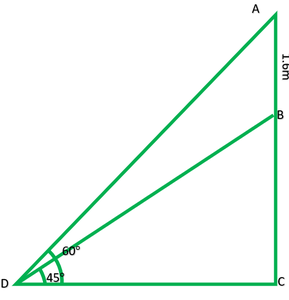### Question 9. The angle of elevation of the top of a building from the foot of the tower is 30° and the angle of elevation of the top of the tower from the foot of the building is 60°. If the tower is 50 m high, find the height of the building.

Solution:

In fig:

AB = tower = 50m

DC = building = ?

In rt.∆ABC,= tan60°

√3 BC = 50

BC = 50/√3

In rt. ∆DCB= tan30°= 1/√3

DC = 50/√3

DC = 50/√3 * 1/√3

DC = 50/3

DC =The height of the building ism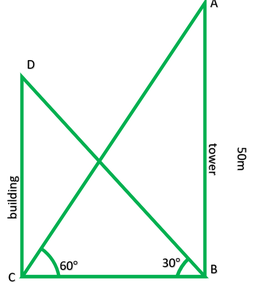### Question 10. Two poles of equal heights are standing opposite each other on either side of the road, which is 80 m wide. From a point between them on the road, the angles of elevation of the top of the poles are 60° and 30°, respectively. Find the height of the poles and the distances of the point from the poles.

Solution:

AB and CD on equal poles.

Let their height = h

Let DP = x

Then PB = BD – x

In rt. ∆CDP,= tan60°

h/x = √3/1

h = √3 x           -(1)

In rt. ∆ABP= tan30°

h/(80 – x) = 1/√3

h = (80 – x)/√3          -(2)

From (1) and (2)

(√3 x)/1 = 80 – x/√3

3x = 80 – x

3x + x = 80

4x = 80

X = 80/4

X = 20

Putting values of X in equation 1

h = √3 x

h = √3(20)

h = 1.732(20)

h = 34.640

Height of each pole = 34.64m

The point is 20m away from first pole and 60m away from second pole.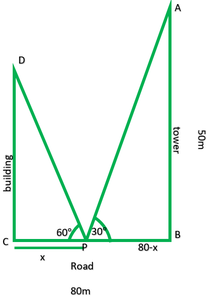### Chapter 9 Some Application of Trigonometry – Exercise 9.1 | Set 2

My Personal Notes arrow_drop_up